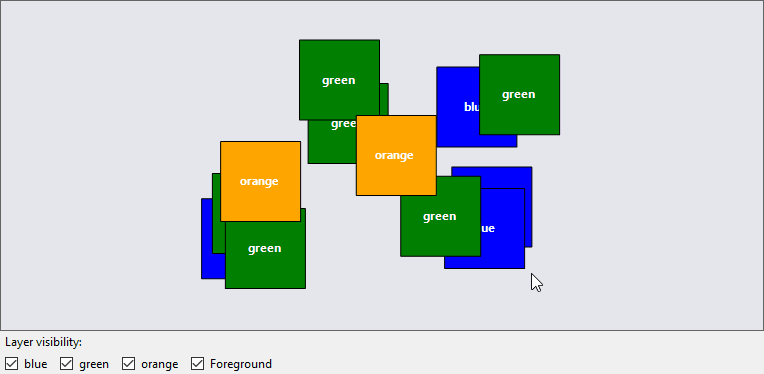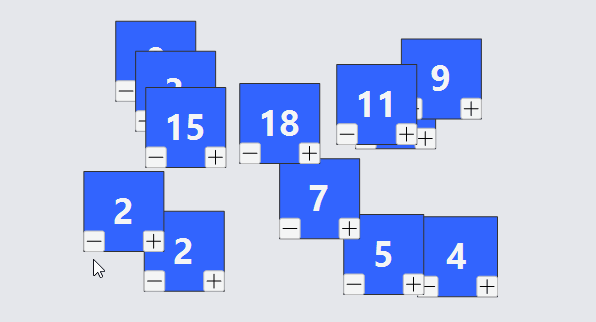# Layers and Z-ordering

All Parts that are in a Diagram actually belong to a Layer in the diagram. You can control the visibility, Z-order, and various user permissions for all of the parts in each layer.

Parts can be individually modified to toggle their visibility using Part.Visible or Part.Opacity. Parts can be individually Z-ordered within layers using Part.ZOrder.

## Standard Layers

Every Diagram starts off with several standard layers. These are their Layer.Names, in order from furthest behind to most in front:

• "Grid", holding the Diagram.Grid and any other static Parts that you wish to be behind everything
• "Background"
• "", the default layer
• "Foreground"
• "Tool", holding Parts used in the execution of various Tools

Each Part is placed in a Layer according to its Part.LayerName. The default value is the empty string. Use Diagram.FindLayer to find a Layer given a layer name. Change which layer a part is in by setting Part.LayerName.

Changes to Parts in the "Grid", "Adornment", and "Tool" Layers are automatically ignored by the UndoManager, because Layer.IsTemporary is true.

Parts in the "Grid" Layer are not selectable, because Layer.AllowSelect is false. This prevents the user from selecting the background grid when it is visible.

## Layers Example

This example adds several Layers to the diagram, each named by a color, and then creates a bunch of colored parts at random locations. Every Part.LayerName is data-bound to the "color" property of the node data.

In addition there are checkboxes for each layer, controlling the visibility of the respective layer. You can see how all of the parts of the same color appear and disappear according to the value of the checkbox. Furthermore you can see how they all have the same depth in the Z-ordering.

Finally, each Part has a Part.SelectionChanged function which puts the part in the "Foreground" layer when it is selected and back in its normal color layer when it is not selected.

``````
// These new layers come in front of the standard regular layers,
// but behind the "Foreground" layer:
var forelayer = diagram.FindLayer("Foreground");
diagram.AddLayerBefore(new Layer { Name = "blue" }, forelayer);
diagram.AddLayerBefore(new Layer { Name = "green" }, forelayer);
diagram.AddLayerBefore(new Layer { Name = "orange" }, forelayer);

diagram.NodeTemplate =
new Part("Spot") {  // no links or grouping, so can use the simpler Part class
SelectionChanged = p => {
p.LayerName = p.IsSelected ? "Foreground" : (p.Data as NodeData).Color;
},
LayerChanged = (p, oldLayer, newLayer) => {
if (newLayer != null) (p.Elt(1) as TextBlock).Text = newLayer.Name;
}
}
.Bind("LayerName", "Color")
.Bind("Location")
new Shape { Width = 80, Height = 80 }
.Bind("Fill", "Color"),
new TextBlock {
Stroke = "white", Font = new Font("Segoe UI", 12, FontWeight.Bold)
}
);

var rand = new Random();
var list = new List<NodeData>();
for (var i = 0; i < 12; i++) {
var data = new NodeData { Location = new Point(rand.NextDouble() * 400, rand.NextDouble() * 200) };
switch (Math.Floor(rand.NextDouble() * 3)) {
case 0: data.Color = "blue"; break;
case 1: data.Color = "green"; break;
case 2: data.Color = "orange"; break;
default: data.Color = "Foreground"; break;
}
}

diagram.Model = new MyModel { NodeDataSource = list };

void toggleVisible(string layerName, bool isChecked) {
diagram.Commit(d => {
var layer = d.FindLayer(layerName);
if (layer != null) layer.Visible = isChecked;
}, "toggle " + layerName);
}

blueCb.CheckedChanged += (s, e) => { toggleVisible("blue", blueCb.Checked); };
greenCb.CheckedChanged += (s, e) => { toggleVisible("green", greenCb.Checked); };
orangeCb.CheckedChanged += (s, e) => { toggleVisible("orange", orangeCb.Checked); };
foregroundCb.CheckedChanged += (s, e) => { toggleVisible("Foreground", foregroundCb.Checked); };
``````## ZOrder Example

This example adds several Parts to one Layer (the default) in the diagram. Every Part.ZOrder is data-bound to the "ZOrder" property of the node data, as is its text.

Buttons on the Part can be used to modify the z-order of each.

``````
void changeZOrder(int amt, GraphObject obj) {
diagram.Commit(d => {
var data = obj.Part.Data as NodeData;
d.Model.Set(data, "ZOrder", data.ZOrder + amt);
}, "modified z order");
}

diagram.NodeTemplate =
new Part("Spot")
.Bind("LayerName", "Color")
.Bind("Location")
.Bind("ZOrder")
new Shape { Width = 80, Height = 80, Stroke = "rgb(50, 50, 50)", Fill = "rgb(50, 100, 255)" },
new TextBlock {
Stroke = "whitesmoke", Font = new Font("Segoe UI", 36, FontWeight.Bold)
}
.Bind("Text", "ZOrder", z => z.ToString()),
Builder.Make<Panel>("Button")
.Set(new {
Alignment = Spot.BottomLeft, AlignmentFocus = Spot.BottomLeft,
Click = new Action<InputEvent, GraphObject>((e, obj) => {
changeZOrder(-1, obj);
})
})
.Add(new Shape("LineH") { Width = 14, Height = 14 }),
Builder.Make<Panel>("Button")
.Set(new {
Alignment = Spot.BottomRight, AlignmentFocus = Spot.BottomRight,
Click = new Action<InputEvent, GraphObject>((e, obj) => {
changeZOrder(1, obj);
})
})
.Add(new Shape("PlusLine") { Width = 14, Height = 14 })
);

var rand = new Random();
var list = new List<NodeData>();
for (var i = 0; i < 12; i++) {
var data = new NodeData { Location = new Point(rand.NextDouble() * 400, rand.NextDouble() * 200) };
data.ZOrder = (int)(rand.NextDouble() * 20);